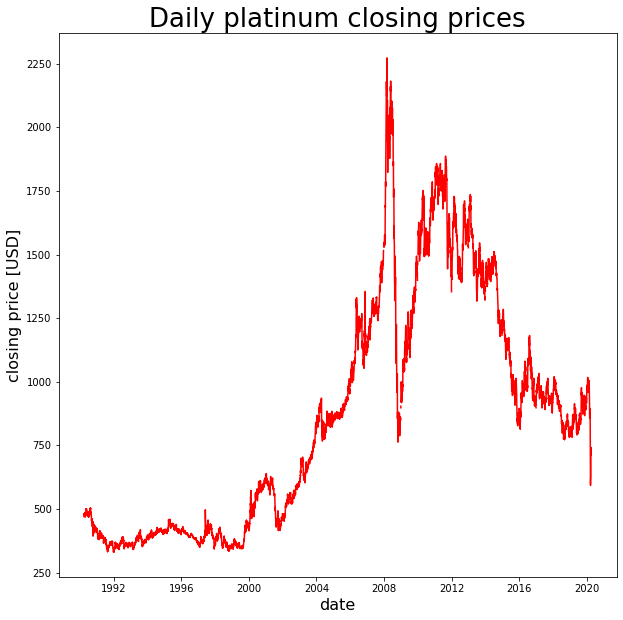# Retrieving platinum and palladium prices from Quandl in PythonIn a previous post I demonstrated how one can query automotive data via Quandl directly from within a Python script.

In this post I will document how to query platinum and palladium exchange prices from Quandl in Python. The data has been verified by London Palladium and Platinum market (http://www.lppm.com).

##### In the code below I retrieve a data set with platinum prices from Quandl
```import quandl
# setting up API key
import numpy
import pandas
# retrieving data from quandl in numpy format, then converting into pandas DataFrame
data = pandas.DataFrame(quandl.get('LPPM/PLAT', returns="numpy"))
```

The data set obtained includes daily opening and closing prices from metal exchange markets around the world. We can take a look at the header of the data frame obtained:

```data.head()
```

As can be seen the daily opening and closing prices are noted in USD, EUR, and GBP. Some of the columns are empty, however. Let us see if this is only the case for early entries by also checking the tail of the data frame retrieved:

```data.tail()
```

For revent days prices were listed for all currencies. Since opening and closing prices were listed in USD both in 1990 and in 2020 I choose to focus on prices in USD only.

##### In the code below I plot platinum closing price history in USD, using matplotlib
```import matplotlib.pyplot as plt
plt.figure(figsize = (10,10))
plt.plot(data["Date"], data["USD PM"],color = "red")
plt.title("Daily platinum closing prices",size=26)
plt.ylabel("closing price [USD]",size = 16)
plt.xlabel("date", size = 16)
```
`Text(0.5, 0, 'date')`
##### In the code below I repeat above workflow for palladium price history

First, I query the data from Quandl:

```data = pandas.DataFrame(quandl.get('LPPM/PALL', returns="numpy"))
```

Next, I review the header of the data frame:

```data.head()
```

And I review the tail of the data frame too:

```data.tail()
```

Again, I choose to focus on closing prices in USD. I plot the price history below, using matplotlib in Python:

```plt.figure(figsize = (10,10))
plt.plot(data["Date"], data["USD PM"],color = "red")
`Text(0.5, 0, 'date')`